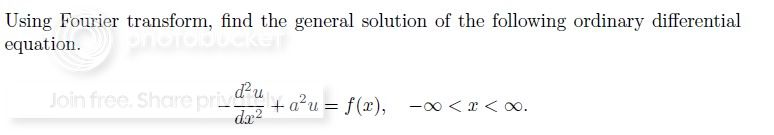fourier transform of DE

Status
Not open for further replies.

naspek

Member level 1hey there..
i've got question on fourier transform..
the question is....i've done until..

\begin{aligned} \widehat{y}=\frac{\widehat{f}}{s^2+a^2}\\ =\widehat{g}\widehat{f}

is this my general solution..?

Last edited by a moderator:

naspek

Member level 1i've done the inverse..

F^-1[g^(k)] = g(x) = 1/ √2∏ ∫g^(k)e^ikx dk
.............................. = 1/ √2∏ ∫ 1/ (k^2 + a^2)e^ikx dk
...............................= √2∏ [(e^-a|x|) / 2a]

what's the next step?

Status
Not open for further replies.